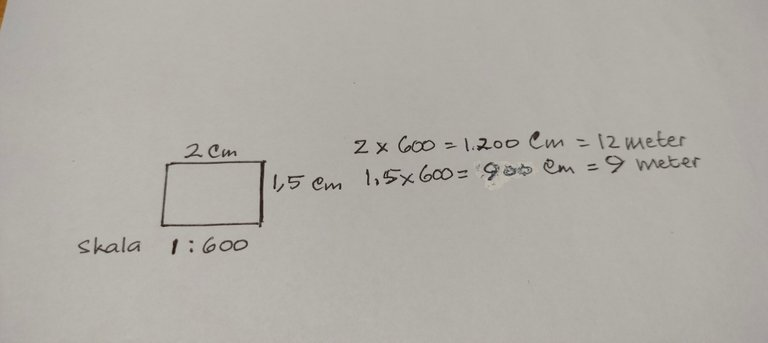# Learn math How to Determine the Image Scale?

in Cent6 months agoImage source
https://www.universitas123.com

Scale is the ratio of the image size to the actual size. Scales are used when someone is going to make a building such as a house or office building. Scale is also used when someone is going to draw a map. Scales are used because it is impossible to make an image of the same size as the original. Someone who is going to make a house or building must have made a design in the form of a picture or what is often called a floor plan. So when drawing this plan we have to use a scale.

For example, if we are going to build a house on land that is 12 meters long and 9 meters wide, it is impossible for us to draw a floor plan on paper that is 12 meters wide and 9 meters wide, so to draw a house we have to use a scale. What scale we will use all depends on ourselves. In simple terms, we can make a scale plan from 1 to 600 or it is often written 1: 600. This means that 1 cm on the map represents 600 cm in actual size. The first step we have to know is that we convert 12 meters into centimeters, that is, 12 meters = 1200 centimeters. And 9 meters = 900 centimeters.If the scale we are going to use is 1:600. So 1,200 centimeters : 600 centimeters = 2 centimeters. And 900 centimeters: 600 centimeters = 1.5 centimeters. So the image we create is like this:

Thank you for dropping by on my blog. This is a trial and error post to practice a little basic math in calculating scales. Hopefully this post is useful.